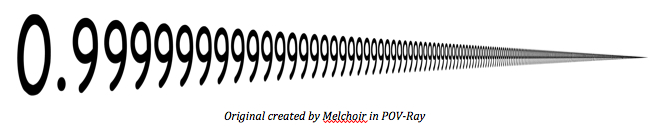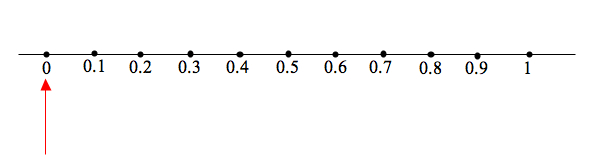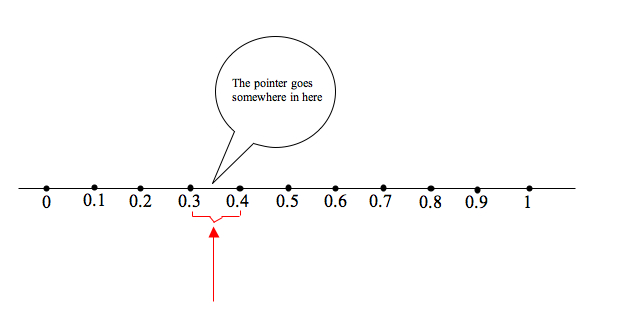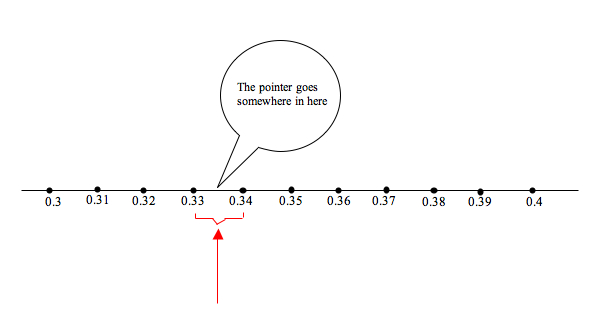# The moving pointer and 0.999…. A useful cognitive root

Posted by: Gary Ernest Davis on: November 1, 2010Debates arise periodically as to whether 0.999… is equal to 1.  Here, the the 9’s never end in the expression “0.999…” .

To paraphrase at least one U.S. President, the answer depends on what we mean by “equal”.

#### Equality

Equality takes some explaining in a mathematical setting.

As an example that is familiar to almost everyone, the fractions$\frac{1}{2}$ and$\frac{500}{1000}$ are commonly regarded as equal and we write$\frac{1}{2}=\frac{500}{1000}$.

Yet$\frac{1}{2}$ is not identical with$\frac{500}{1000}$. There are least two ways in which this is so. First, we do not see a$500\textrm{ or } 1000$ in the fraction$\frac{1}{2}$. The form of these two fractions is different. Secondly, 1 part of an object – such as a cupcake – that has been divided into 2 equal pieces is quite different from 500 pieces of a cupcake that has been divided into 1000 equal pieces.

So what do we mean when we say that$\frac{1}{2}$ and$\frac{500}{1000}$ are equal?

We mean that$1\times 1000=2\times 500$.

More generally, two fractions$\frac{a}{b}, \frac{c}{d}$ are equal when$a\times d = b\times c$. Here, we take it for granted that we know what we mean by equality of whole numbers.

#### Decimals

Fractions can be written as – possibly infinite – decimal strings using the digits$0, 1, \ldots 9$.

The basic idea of a decimal string$x=0.a_1a_2a_3\ldots$ is that multiplication by 10 moves the digits to the left:$10\times x = a_1+0.a_2a_3a_4\ldots$. The reason for this is that we are thinking – in the back of our minds – that$x=0.a_1a_2a_3\ldots$ should mean$\frac{a_1}{10}+\frac{a_2}{100}+\frac{a_3}{1000}+\ldots$, whatever a possibly infinite sum might mean.

We avoid thinking about infinite sums by just focusing on the decimal strings themselves.

How could we write a fraction such as$\frac{1}{3}$ as a decimal string:$\frac{1}{3}=0.a_1a_2a_3\ldots$?

Using the basic property of decimal strings, described above, we would have$10\times\frac{1}{3}=a_0+0.a_2a_3a_4\ldots$. But$10\times\frac{1}{3} = 3+\frac{1}{3}$ so we would have$a_1=3 \textrm{ and } 0.a_2a_3a_4\ldots =\frac{1}{3}$. Now we are right back where we started, so we will have$a_n=3\textrm{ for all } n$.

In other words, using the basic property of decimal strings that multiplication by 10 moves the digits to the left, we have the  decimal representation$\frac{1}{3}=0.333\ldots$. Here the “3”s go on forever, without end.

This is a cute trick, but what could it mean? We cannot resort to infinite sums if we do not know what real numbers are, so we need another interpretation to guide our intuition. This is not so much a matter of mathematical logic, which is well-covered by any number of ways of constructing the real numbers, as it is of an intuitive understanding of equality of real numbers, represented as decimal strings. Our aim is to increase, not decrease, understanding for students and teachers.

#### A decimal string as a sequence of instructions

Let’s think about a decimal string$0.a_1a_2a_3\ldots$ as a sequence of instructions: each of the digits$a_n$ is instructing us to move a pointer to a certain place on a line. We will use the decimal representation$0.333\ldots \textrm{ for } \frac{1}{3}$ to illustrate what we mean by a sequence of instructions for moving a pointer on a line.

Imagine a pointer pointing at 0 on the number line:Here the decimal strings$0.1, 0.2, \ldots 0.9$ have been placed on the line at spots that divide the numbers between 0 and 1 into 10 equal-sized intervals. These decimal strings represent the numbers$\frac{1}{10},\frac{2}{10}, \ldots , \frac{9}{10}$.

The first digit – namely 3 – in the decimal string for$\frac{1}{3}$ indicates that we should move the pointer somewhere between the$0.3 \textrm{ and} 0.4$ spots:To figure out where between 0.3 and 0.4 the pointer goes, we divide the space between 0.3 and 0.4 into 10 equal size intervals. We label the new endpoints of these intervals$0.31, 0.32, 0.33, 0.34, 0.35, 0.36, 0.37, 0.38, 0.39$.The second digit – namely 3, again – in the decimal string for$\frac{1}{3}$ indicates that we should move the pointer somewhere between the$0.33 \textrm{ and} 0.34$ spots, as in the diagram.

Again, this does not pin down the pointer exactly. So we take the interval between 0.33 and 0.34 and divide it into 10 equal sized intervals. The new labels for the endpoints of these intervals will be$0.331, 0.332, 0.333, 0.334, 0.335, 0.336, 0.337, 0.338, 0.339$.

We read the third  digit-  3, again – in the decimal string for$\frac{1}{3}$ and move the pointer somewhere between the$0.333 \textrm{ and} 0.334$ spots.

This process of moving the pointer will never end, because there is a never ending sequence of 3’s in the decimal string for$\frac{1}{3}$. The pointer never stops: it never actually points at$\frac{1}{3}$ at any stage we move it.

In terms of the moving pointer, how can we interpret$\frac{1}{3}=0.333\ldots$?

One way to answer this question is to say that as we move the pointer according to the instructions encoded in the decimal string$0.333\ldots$, the distance between the pointer and$\frac{1}{3}$ becomes progressively closer to 0.

If we had placed the pointer at the left hand end of an interval at each step, the distance between the pointer and$\frac{1}{3}$ would reduce by$\frac{1}{10}^{th}$ each time.

That it is one way to think about the “=” in$\frac{1}{3}=0.333\ldots$.

This is what is known as a “cognitive root” in the learning and teaching of mathematics:

• It is an idea that is based on something relatively easy to grasp.
• It is not the whole story – more sophisticated mathematical thought adds to, and enriches the idea.
• The idea is not thrown away as a student encounters more sophisticated mathematics: it is simply built upon.

The same idea of “equals” applies to$0.999\ldots = 1$.

We imagine a pointer located at 0 and move it to 0.9. The distance between the pointer and 1 is$\frac{1}{10}$.

We take the next digit in the decimal string – 9 – and move the pointer to 0.99. The distance between the pointer and 1 is now$\frac{1}{100}$. Each time we move the pointer according to the instruction encoded in the next digit in the decimal string the distance between the pointer and 1 is reduced by$\frac{1}{10}^{th}$.

That is the sense in which we mean “=” in$0.999 \ldots = 1$.

It all depends,  to paraphrase a former US President again, what we mean by “equals.”

For a related discussion, see James Tanton’s video “Point Nine Forever”:

James rightly points out, in different terminology,  that if the decimal string$x = 0.999\ldots$ is to represent a number at all, then, by our fundamental operation of multiplying the decimal string by 10 we have$10\times x = 9+0.999\ldots = 9+x \textrm{ so } 9\times x = 9 \textrm { and therefore } x=1$

### 7 Responses to "The moving pointer and 0.999…. A useful cognitive root"Wonderful article!Thanks. Odd the link is broken. It seemed OK when I clicked on it. Here’s the URL: http://www.warwick.ac.uk/staff/David.Tall/themes/cognitive-roots.html“So what do we mean when we say that 1/2 and 500/1000 are equal?”

Just being completely picky, as usual Gary, I would never say that! Equivalent, maybe, but “equal” no!

Re: 0.999999999999… It’s like asking whether the sum of (1/2)^n for all values of n from 1 to infinity is equal to 1… (of course it tends to 1 as n tends to infinity, but that is different!).

Further, what if 1/3 were represented using binary instead of decimal fractions…

What is usually missed in most of these discussions is that recurring decimals can be represented by rational fractions, whereas non-recurring cannot. That, to me, is the more interesting mathematics!

ColinColin, you may say equivalent, but most elementary teachers say “equal”.

Equality is a very strange notion. No two distinct things can be equal, else they would not be distinct. So almost all “equality” is equivalence. The only distinguishing thing about equality is that it is a symmetric, transitive, reflexive relationship: that is, an equivalence relation.

I agree with you about the non-recurring decimals, but that’s a story for another post. Would you like to write it?jfW767 Very true! Makes a change to see someone spell it out like that. :)Here’s another way to think about it. Two real numbers are equal if their difference is 0. Working one digit at a time, the difference between these two numbers is easily seen to be less than 0.1, less than 0.01, less than 0.001, and so on. Hence, it is smaller than 1/10^n for any positive integer n, and smaller than any positive number. Hence, the difference is 0, unless you want to try to argue that 0.99999… > 1, which is shown to be false merely by looking at the ones digit of each number.My experience with undergraduates is that they believe in infinitesimals. Many of them would not accept that just because a non-negative number is less then$\epsilon$ for arbitrarily small$\epsilon$ it must be 0.